[′rād·ē·ō ¦wāv ‚präp·ə‚gā·shən]
(electromagnetism)
The transfer of energy through space by electromagnetic radiation at radio frequencies.
McGraw-Hill Dictionary of Scientific & Technical Terms, 6E, Copyright © 2003 by The McGraw-Hill Companies, Inc.
The following article is from The Great Soviet Encyclopedia (1979). It might be outdated or ideologically biased.

the propagation of radio-frequency electromagnetic waves in the atmosphere, in outer space, and underground. The path traveled by radio waves between a transmitter and a receiver may be complicated. The waves may reach the receiving point by, for example, propagating along straight-line paths, bending around the curved surface of the earth, or being reflected from the ionosphere. The manner in which radio waves propagate depends on a number of factors, such as the wavelength λ and the intensity of the sun’s illumination of the earth’s atmosphere.

Direct waves. In homogeneous media, radio waves propagate rectilinearly at a constant speed like light rays, and we may thus speak of radio rays. Such propagation is said to be free. Nearly free propagation occurs in several cases—for example, in radio communication between an earth station and a space vehicle or between two space vehicles, in radio-astronomical observations, and in radio communication between an earth station and an aircraft or between two aircraft.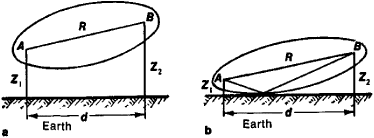Figure 1. Region of importance in the propagation of radio waves: (A) transmitting antenna, (B) receiving antenna, (Z1) and (Z2) heights of the antenna above the earth’s surface

A wave at great distances from the antenna that radiated it can be regarded as a plane wave. The flux density of the electromagnetic energy is proportional to the square of the field strength of the wave. Since the flux density decreases with increasing distance r from the source in inverse proportion to r2, the distance at which the signal from a transmitting station can be received is limited. The transmission range of a radio station is, in the absence of absorption, equal towhere Ps is the power of the signal at the receiver input, Pn is the power of the noise, and G1 and G2 are the directive pattern gains of the transmitting and receiving antennas, respectively. The propagation speed of radio waves in free space is equal to the speed of light in a vacuum: c = 300,000 km/sec.

When a radio wave propagates in a material medium such as the atmosphere, the upper layer of the earth, or seawater, its phase velocity is changed, and energy is absorbed. These effects occur because the electric field of the wave causes oscillations of the electrons and ions in the atoms and molecules of the medium, and these particles reradiate secondary waves. If the field strength of the wave is small compared with the strength of the field acting on an electron in an atom, then the electron oscillates harmonically at the frequency of the passing wave. Consequently, the electrons emit radio waves of the same frequency but with different amplitudes and phases. The phase shift between the primary and secondary waves causes a change of the phase velocity. The energy losses that occur when radio waves interact with atoms are responsible for the absorption of the waves. The absorption and the change of the phase velocity in a medium can be described by the extinction coefficient κ and the index of refraction n, which, in turn, depend on the dielectric constant ∊ and the conductivity σ of the medium and on the wavelength λ: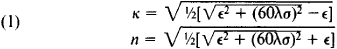The absorption coefficient is β = 2πκ/λ, and the phase velocity is v = c/n. In this case, rt is determined by the properties of the medium (∊ and σ) as well as by the characteristics of the transmitter and receiver and by the wavelength. The propagation of radio waves under earth conditions is usually different from free propagation. It is influenced by, for example, the earth’s surface, the earth’s atmosphere, and the structure of the ionosphere. The nature of the influence of these factors depends on the wavelength

Influence of the earth’s surface. The influence of the earth’s surface on radio-wave propagation depends on the position of the transmission path with respect to the surface.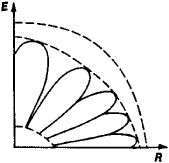Figure 2. Lobed structure of the field at the receiving point

The propagation of radio waves is a three-dimensional process that covers a large region. The most important role in this process, however, is played by the portion of space bounded by a surface with the shape of an ellipsoid of revolution in which the transmitter and receiver are located at the focuses A and B (Figure 1). The major axis of the ellipsoid is practically equal to the distance R between transmitter and receiver, and the minor axis is ~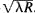. The smaller the wavelength, the narrower the ellipsoid. In the optical range it degenerates into a straight line, and we have a light ray. Suppose the transmitting and receiving antennas are located at the heights Z1 and Z2 above the surface of the earth. If Z1 and Z2 are large compared with λ, then the ellipsoid does not touch the earth’s surface (Figure 1,a). In this case, the earth’s surface does not influence the propagation, which is thus free. When one or both of the end points of the radio-wave path are lowered, the ellipsoid touches the earth’s surface (Figure l,b), and the field of a reflected wave is superposed on the direct wave that goes from the transmitter to the receiver. If, in addition, Z, ≫ λ and Z2λ, then this field can be regarded as a ray that is reflected from the earth’s surface in accordance with the laws of geometrical optics. The field at the receiving point depends on the interference between the direct and the reflected waves. The interference maxima and minima cause the field to have a lobed structure (Figure 2). The condition that Z1 and Z2λ can be met in practice only for wavelengths less than 1 m; consequently, the lobed field structure is typical for meter and decimeter waves.

As λ increases, the region of importance is enlarged and intersects the earth’s surface. When this occurs, the wave field can no longer be represented as the result of the interference of direct and reflected waves. The influence of the earth on the propagation of the radio waves is here due to several factors: the earth has considerable electrical conductivity, and radio-wave propagation along the surface of the earth therefore results in heat losses and the attenuation of the waves. The energy losses in the earth increase as λ decreases.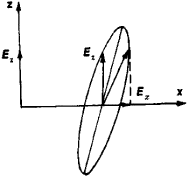Figure 3

In addition to attenuation, there also occurs a change in the structure of the wave field. If an antenna at the earth’s surface radiates a transverse linearly polarized wave, for which the electric field strength E is perpendicular to the earth’s surface, then at large distances from the radiator the wave becomes ellipti-cally polarized (Figure 3). The magnitude of the horizontal component Ex is substantially less than that of the vertical component Ez and decreases as the conductivity σ of the earth’s surface increases. The existence of a horizontal component permits the ground waves to be received by what are called ground antennas—two conductors located on the earth’s surface or at a small height. Suppose an antenna radiates a horizontally polarized wave, for which E is parallel to the earth’s surface. Then the greater the value of σ, the more the earth’s surface attenuates the field, and a vertical component is created. Even at short distances from a horizontal radiator the vertical component of the field is greater than the horizontal component. As the ground waves propagate along the earth, their phase velocity varies with distance. Already at a distance of several λ’s from the radiator, however, the phase velocity becomes equal to the speed of light, regardless of the electrical properties of the soil.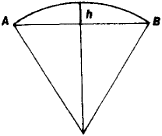Figure 4. Cross section of the spherical segment whose altitude h characterizes the curvature of the earth

The curvature of the earth constitutes a special kind of obstacle in the path of radio waves, which, by diffraction, bend around the earth and enter the shadow zone. Diffraction of waves becomes appreciable when the dimensions of an obstacle are commensurate with or less than λ. The amount of the earth’s curvature can be characterized by the altitude h (Figure 4) of the spherical segment cut off by the plane that passes through the chord connecting the points where the transmitter and receiver are located (see Table 1). It follows that the condition h ≤ λ is met for meter and longer waves. As λ decreases, however, the energy losses in the earth are increased. Thus, es-entially only kilometer and longer waves can penetrate deep into the shadow zone (Figure 5).

Table 1. Altitude ft of the spherical segment for various distances between transmitter and receiver
Distance (km)h(m)
10.03
50.78
103.1
5078
100310
5007,800
1,0003.1 × 104
5,0003.75 × 105

The earth’s surface is inhomogeneous, and the electrical properties of the portions of the transmission path that are adjacent to the transmitter and receiver have the most important influence on the propagation of radio waves. If the transmission path intersects a coastline—that is, if it passes over dry land and then over seawater (σ → ∞)—the field strength changes abruptly when the path crosses the coastline (Figure 6). In other words, the amplitude and the direction of propagation of the wave are altered. We speak in this case of coastline refraction. Coastline refraction, however, is a local disturbance of the radio wave’s field and diminishes as the distance from the coastline increases.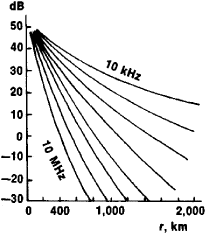Figure 5. Variation of field strength with distance r. The vertical axis shows the attenuation factor; it is defined as the ratio of the field strength under actual propagation conditions to the field strength for propagation in free space.

The topography of the earth’s surface also affects the propagation of radio waves. The influence of topography depends on the relationship between the height of the surface irregularities h, the horizontal extension l of the irregularities, λ, and the angle of incidence θ of the wave on the surface (Figure 7). If the conditions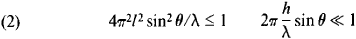are satisfied, then the irregularities are considered to be small and mildly sloping, and they have little effect on radio-wave propagation. When θ is increased, the conditions (2) may be violated. In this case, diffuse reflections occur: the energy of the wave is scattered, and the field strength in the direction of the reflected wave is reduced.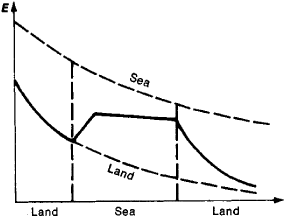Figure 6. Change in the field strength E of a wave when the wave crosses a coastline

In addition, such irregularities as high hills and mountains greatly disturb the field and create shadow zones. The diffraction of radio waves by mountain ranges sometimes results in the reinforcement of the waves because of the interference between the direct waves and waves reflected from the earth’s surface (Figure 8).

Propagation in the troposphere. REFRACTION. Ground radio waves propagate along the earth’s surface through the troposphere. The conductivity σ of the troposphere for frequencies that correspond to radio waves (except for millimeter waves) is practically equal to zero. The dielectric constant ∊ and, consequently, the index of refraction n are functions of the air pressure, air temperature, and water-vapor pressure. At the earth’s surface, n ≈ 1.0003. The variation of ∊ and n with altitude depends on the meteorological conditions. Usually ∊ and n decrease, and the phase velocity v increases, with increasing altitude. Bending, or refraction, of radio waves results (Figure 9). Suppose a wave is propagating in the troposphere at an angle to the horizon and has a wave front that coincides with the straight line ab (Figure 9). Since the wave’s speed of propagation is higher in the upper layers of the troposphere than in the lower layers, the upper part of the wave front overtakes the lower part, and the wave front is tilted—that is, the beam is bent. Because n decreases with increasing altitude, radio beams are deflected toward the earth. This phenomenon is called normal tropospheric refraction. It permits the propagation of radio waves beyond the limits of line-of-sight propagation because the refraction allows the waves to bend around the curvature of the earth. In practice, however, this effect can play a role only for ultrashort waves, since for longer waves bending as a result of diffraction is predominant. Meteorological conditions can result in refraction weaker or stronger than normal.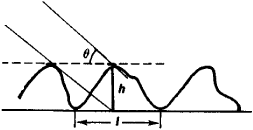Figure 7

TROPOSPHERIC WAVE GUIDE. Under some conditions, such as when heated air moves from the land over the surface of the sea, the air temperature does not decrease with altitude but increases—that is, a temperature inversion occurs. In this case, tropospheric refraction can become so strong that a wave traveling at a small angle to the horizon will change its direction at some altitude and be returned to earth. In the space bounded below by the earth and above by, as it were, a tropospheric reflecting layer, a wave can propagate over very long distances, and we can speak of the wave-guide propagation of radio waves. As in metal wave guides for radio waves, in tropospheric wave guides there can propagate waves whose wavelength is less than the critical wavelength λcr ≈ 0.085d/3/2, where d is the height of the wave guide in m and λcr is in cm. The thickness of an inversion layer in the troposphere is usually not greater than ~50 to 100 m; consequently, only decimeter, centimeter, and shorter waves can propagate by the waveguide method.

SCATTERING FROM FLUCTUATIONS IN ∊. Besides the regular variations in ≪ with altitude, there occur irregular inhomogeneities, or fluctuations, of ∊ as a result of random air motion. Ultrashort radio waves are scattered by these inhomogeneities. Thus, the spatial region bounded by the radiation patterns of the receiving and transmitting antennas and containing a large number of inhomogeneities of ∊ constitutes a scattering volume. The scattering leads to fluctuations in the amplitude and phase of a radio wave and to the propagation of ultrashort waves over distances that substantially exceed the distances for line-of-sight propagation (Figure 10). Here, the field at the receiving point B is formed as the result of the interference of scattered waves. Because of the interference of the large number of scattered waves, random variations in the signal amplitude and phase occur. The average value of the signal amplitude, however, is considerably greater than the value that could result from normal tropospheric refraction.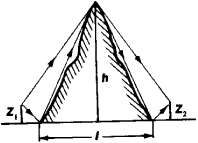Figure 8. Reinforcement of radio waves through diffraction by abrupt irregularities

ABSORPTION. The troposphere is transparent for centimeter and longer waves. Shorter waves undergo marked attenuation by droplet formations, such as rain, hail, snow, and fog, and by water vapor and by atmospheric gases. The attenuation is due to absorption and scattering. Each drop of water has appreciable conductivity, and a wave excites high-frequency currents in the drop. Since the current density is proportional to the frequency, appreciable currents and, consequently, heat losses occur only in the propagation of centimeter and shorter waves. These currents not only give rise to heat losses but are sources of secondary scattered radiation, which weakens the direct signal. The flux density of the scattered energy is inversely proportional to λ4 when the diameter d of the scattering particle is less than λ; the flux density is independent of λ when d ≫ λ. In practice, waves having λ < 3 cm are not propagated through a region of heavy rain or dense fog. Moreover, waves shorter than 1.5 cm undergo resonance absorption in water vapor (λ = 1.5 cm, 1.35 cm, 0.75 cm, 0.5 cm, and 0.25 cm) and in oxygen (λ = 0.5 cm and 0.25 cm). In this case, the energy of the propagating wave is expended in ionizing or exciting atoms and molecules. Between the resonance lines there are regions of low absorption.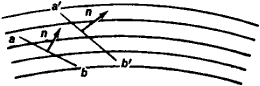Figure 9. Bending of radio waves in the troposphere as a result of the troposphere’s inhomogeneity

Propagation in the ionosphere. In the ionosphere, which is a multicomponent plasma located within the earth’s magnetic field, the mechanism of radio-wave propagation is more complicated than in the troposphere. Under the action of radio waves in the ionosphere there may occur both forced oscillations of electrons and ions and various kinds of collective natural oscillations called plasma oscillations. One of these types plays the principal role, depending on the frequency ω of the radio wave. Consequently, the electrical properties of the ionosphere are different for different frequency ranges. When the frequency ω is high, only electrons participate in the propagation of radio waves, and the natural frequency of their oscillations—the Langmuir frequency—is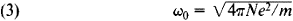Here e is the charge and m the mass of the electron, and N is the electron concentration. The forced oscillations of free electrons in the ionosphere lag behind the electric field of the high-frequency wave by a phase shift of almost 2π; this behavior differs from that of electrons in the troposphere, which are tightly bound to atoms. This displacement of the electrons in the ionosphere reinforces the field E of the wave (Figure 11). The dielectric constant ∊, which is equal to the ratio of the external field strength to the field strength inside the medium, is consequently less than 1 for the ionosphere: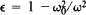. When collisions of the electrons with the atoms and ions are taken into account, more accurate formulas are obtained for ∊ and σ in the ionosphere: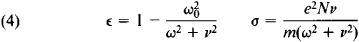where v is the number of collisions per second.

For frequencies higher than 3 megahertz (MHz), the relationship ω2v2 is valid in a larger portion of the ionosphere. For the index of refraction n and the extinction coefficient κ, in this case we have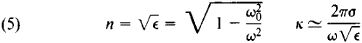As the frequency increases, κ is reduced and n increases, approaching unity. Since n < 1, the phase velocity of propagation of the wave vph = c/n > c. The propagation velocity of the energy (the group velocity of the wave) in the ionosphere is equal to cn; in accordance with relativity theory, the group velocity is less than c.

REFLECTION. For a wave such that ω < ω0, n and v are imaginary quantities. This means that the wave cannot be propagated in the ionosphere. The electron concentration N and the plasma frequency ω0 in the ionosphere increase with increasing altitude (Figure 12). Thus, when an incident wave enters the ionosphere, it propagates to the level where the index of refraction becomes zero. At this altitude, the wave is totally reflected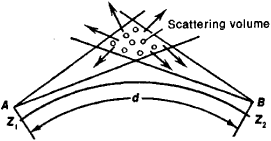Figure 10. Schematic representation of a radio link that makes use of the scattering of radio waves by inhomogeneities in the troposphere

from the ionospheric layer. As the frequency increases, the incident wave penetrates deeper and deeper into the ionospheric layer. The maximum frequency of the wave that is reflected at vertical incidence from an ionospheric layer is called the critical frequency of the layer: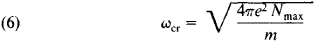The critical frequency of the F2 layer (the principal maximum in Figure 12) varies in the course of the day and from year to year between approximately 5 and 10 MHz. For waves having frequency ω > ωcr, n is everywhere greater than zero—that is, the wave passes through the layer without being reflected.

For a wave obliquely incident on the ionosphere, the maximum frequency at which the wave is returned to the earth is higher than ωcr. A radio wave that is incident on the ionosphere at an angle ϕ0 undergoes refraction and is returned to the earth from the altitude where ϕ(z) = π /2. The reflection condition for oblique incidence has the form n(z) = sin ϕ0. The frequencies of the waves that are reflected from a given altitude at oblique and vertical incidence are connected by the relation ωobl = ωvert sec ϕ0. The maximum frequency at which a wave is reflected from the ionosphere for a given angle of incidence, that is, for a given distance between transmitter and receiver, is called the maximum usable frequency (MUF).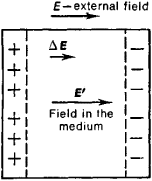Figure 11. The displacement of electrons in the ionosphere under the action of a wave field E results in the appearance of an additional field ΔE

DOUBLE REFRACTION. The earth’s magnetic field H0 = 0.5 oersted, which permeates the ionosphere, has an important influence on the propagation of radio waves. In a constant magnetic field, an ionized gas becomes an anisotropic medium. Upon entering the ionosphere, a wave undergoes double refraction; that is, it is split into two waves that have different speeds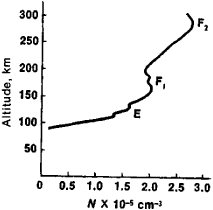Figure 12. Variation of the electron concentration N in the ionosphere with altitude; (E), (F1), and (F2) layers in the ionosphere

and directions of propagation, different absorptions, and different polarizations. An electron moving in a magnetic field H0 at a velocity v is subject to the Lorentz force F = e/c [vH0], which causes the electron to revolve at the gyrofrequency ωH = eH0/mc about the lines of force of the magnetic field. As a result, the nature of the electron oscillations induced in the ionosphere by the electric field of a wave is changed.

In the simple case where the direction of propagation is perpendicular to H0—that is, E lies in the same plane as H0—the wave can be represented as the sum of two waves with E ⊥ H0 and EǀǀH0. For the first wave, called the extraordinary wave, the nature of the electron motion and, therefore, of n changes; for the second wave, called the ordinary wave, the electron motion and n remain the same as when no magnetic field is present: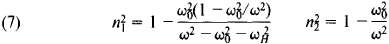When the direction of propagation relative to the earth’s magnetic field is arbitrary, the formulas are more complicated: both n1 and n2 depend on ωH. Since the reflection of a radio wave occurs at the layer where n = 0, the ordinary and the extraordinary waves are reflected at different altitudes. Their critical frequencies also differ.

As the ordinary and extraordinary waves propagate in the ionosphere, the phase shift between them continually increases because of the velocity difference. Consequently, the polarization of the resultant wave changes continually. Under certain conditions, the linear polarization of the incident wave is preserved, but the plane of polarization is rotated during the propagation. In the general case, the polarization of both waves is elliptical.

SCATTERING. In addition to the regular variation of the electron concentration N with altitude (Figure 12) in the ionosphere, random variations of the concentration are constantly occurring. An ionospheric layer contains a large number of irregular formations of different sizes that are in a state of constant motion and change—being resorbed and then reappearing. As a result, in addition to the principal reflected signal, a great number of scattered waves arrive at the receiving point (Figure 13). The superposition of these waves results in fading, that is, random variations in signal intensity.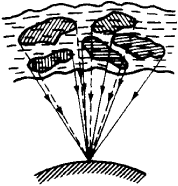Figure 13. Scattering of radio waves by nonuniformities in the ionosphere

The existence of the nonuniformities makes possible the diffuse reflection of radio waves at frequencies substantially higher than the maximum frequencies for reflection from the regular ionosphere. Like scattering by inhomogeneities in the troposphere, this phenomenon is responsible for the propagation of radio waves (in the meter range) over longer distances.

Typical nonuniformities occur in the ionosphere when meteors enter it. The electrons emitted by an incandescent meteor ionize the surrounding medium and form behind the meteor a trail, whose diameter increases rapidly owing to molecular diffusion. The ionized trails are produced at an altitude of 80 to 120 km, and they exist for 0.1 to 100 sec. Radio waves are reflected specularly from a meteor trail. The effectiveness of this process depends on the mass of the meteor.

NONLINEAR EFFECTS. For signals of moderate power, two radio waves can propagate through the same region of the ionosphere independently of each other—that is, the ionosphere is a linear medium. For powerful radio waves, where the field E of the wave is comparable with the characteristic plasma field Ep of the ionosphere, ∊ and σ show a dependence on the field strength of the propagating wave. The linear relationship between the electric current and the field E is violated.

The nonlinearity of the ionosphere can show up in the Luxembourg effect—that is, the cross modulation of two signals—or in the “self-reaction” of a high-power wave, for example, in a change in the depth of modulation of a signal that is reflected from the ionosphere.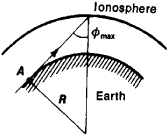Figure 14

SPECIAL FEATURES OF THE PROPAGATION OF RADIO WAVES OF DIFFERENT FREQUENCY RANGES IN THE IONOSPHERE. Ultrashort waves, whose frequencies are higher than the MUF, pass through the ionosphere. Waves with a frequency below the MUF are reflected from the ionosphere and return to the earth. Such waves are called ionospheric, or sky, waves and are used for long-range radio communication on the earth. The frequency range of ionospheric waves is limited on its low-frequency side by absorption. Communication by means of ionospheric waves consequently takes place in the high-frequency band and, at nighttime (when the absorption is reduced), in the medium-frequency band. When one reflection from the ionosphere occurs, the transmission range is ~3,500–4,000 km, since the angle of incidence ϕ on the ionosphere is limited by the curvature of the earth: at the maximum angle of incidence the ray is tangent to the earth’s surface (Figure 14). For longer-range communication, several reflections from the ionosphere are necessary (Figure 15).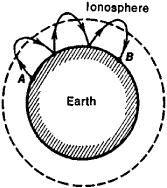Figure 15

Waves of frequency less than 300 kilohertz (kHz) are essentially unable to penetrate the ionosphere and are reflected from its lower boundary. This lower boundary forms, as it were, the wall of a spherical radio wave guide whose second wall is the earth. Waves radiated by an antenna at a point on the earth bend around the earth in all directions and converge on the opposite side. Superposition of the waves causes an increase in the field strength at the opposite point, a phenomenon called the antipodal effect (Figure 16).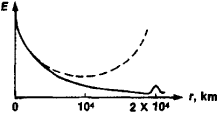Figure 16. Field strength E of a wave as a function of the distance r to the transmitter when no absorption occurs (broken line) and when absorption is taken into account

Audio-frequency radio waves can penetrate through the ionosphere along the lines of force of the earth’s magnetic field. When the waves propagate along a line of force, they go out to a distance equal to several earth radii and then return to the conjugate point in the opposite hemisphere (Figure 17). Lightning discharges in the troposphere are a source of such waves. When the waves propagate in the manner described, they produce whistling sounds in the output of a receiver, which are called whistlers, or whistling atmospherics.

For infrasonic-frequency radio waves, whose frequency is lower than the gyrofrequency of the ions, the ionosphere acts like a neutral conducting fluid whose motion can be described by the equations of hydrodynamics. Owing to the presence of the earth’s magnetic field, any displacement of a conducting substance whereby an electric current is produced is accompanied by the appearance of Lorentz forces, which alter the state of the motion. The interaction between the mechanical and electromagnetic forces leads to the transference of a randomly occurring movement in the ionized gas into a motion along the magnetic lines of force—that is, to the appearance of magneto-hydrodynamic waves. Called Alfvén waves, these magnetohy-drodynamic waves propagate along the magnetic lines of force with a speed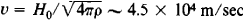, where ρ is the density of the ionized gas.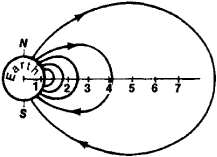Figure 17

Space radio communication. When a receiver or transmitter is located on the earth, the band of wavelengths suitable for communication with a space vehicle is determined by the conditions for transmission through the earth’s atmosphere. As we have seen, radio waves having a frequency less than the MUF, which ranges from 5 to 30 MHz, do not pass through the ionosphere. Waves having a frequency greater than 6 to 10 gigahertz (GHz) are absorbed in the troposphere. Thus, waves from a space vehicle can be received on the earth at frequencies from about 30 MHz to 10 GHz. The earth’s atmosphere, however, is not completely transparent for radio waves even in this range. When the waves are received by an ordinary antenna, the rotation of their plane of polarization that occurred in their passage through the ionosphere results in losses that decrease as the frequency increases. These losses are negligible only at frequencies higher than 3 GHz (Figure 18). These conditions determine the wave band for long-range communication with satellites as metric or decimetric waves.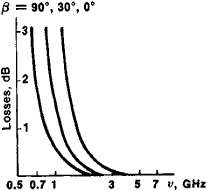Figure 18. Energy losses due to the rotation of the plane of polarization of a wave as a function of frequency for three values of the angle of elevation β

In order to communicate with other planets, it is necessary to take into account absorption also in the atmospheres of these planets. Millimeter waves and light waves are of particular importance for communication between two space vehicles that are outside planetary atmospheres. Such waves provide the largest communication-channel capacity. Information on the propagation processes of radio waves in outer space is provided by radio astronomy.

Underground and underwater radio communication. The earth’s crust and the water of the seas and oceans have electrical conductivity σ and strongly absorb radio waves. For sedimentary rocks in the surface layer of the earth’s crust, σ ≈ 10–3–10–2 (ohm-m)–1. In such media, a wave is practically damped out at distances ≤ λ. Moreover, in media with a large σ the absorption coefficient increases with frequency. Therefore, primarily frequencies less than 300 kHz are used for underground radio communication. In underwater communication, waves in the optical band are used along with waves of frequency less than 30 kHz.

In communication systems between underground or underwater points, it may be possible to make use of propagation partly along the surface of the earth or sea. A vertically polarized wave excited by an underground transmitting antenna propagates up to the earth’s surface, is refracted at the interface between the earth and the atmosphere, propagates along the earth’s surface, and is then received by an underground receiving antenna (Figure 19). The depth at which antennas are located can reach tens of meters. Systems of this type have a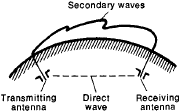Figure 19. Underground communication system with propagation of the radio waves partly along the earth’s surface; the secondary waves are depicted in arbitrary fashion

range of up to several hundred km and are made use of, for example, in communication between underground control points during the launching of a rocket. Another type of system makes use of underground wave guides—layers of the earth’s crust that have low conductivity and, as a result, low losses. Such layers may consist of, for example, rock salt or potash. The layers lie at depths as great as hundreds of meters and provide a transmission range of up to several tens of km. A further development in this direction is the use of hard rocks, such as granites, gneisses, and basalts, that are located at great depths and have low conductivity (Figure 20). At depths of 3 to 7 km, σ may be reduced to 10–11 (ohm-m)-1. With a further increase in depth, the rising temperature produces ionization (an inverted ionosphere), and the conductivity increases. An underground wave guide is formed that has a thickness of several km. Radio waves can propagate over distances of up to several thousand km through such a wave guide. One of the fundamental problems of underground and underwater communication is the calculation of the radiation and energy transfer from antennas located in a conducting medium.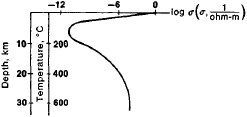Figure 20. Variation of conductivity σ of the earth with depth

Underground communication systems have the advantage of not being subject to the storms, hurricanes, and man-made disturbances on the earth’s surface. In addition, because of the shielding effect of the upper conducting sedimentary rocks, underground communication systems are well protected against industrial and atmospheric interference.

### REFERENCES

Feinberg, E. L. Rasprostranenie radiovoln vdol’ zemnoi poverkhnosti. Moscow, 1961.
Al’pert, la. L. Rasprostranenie elektromagnitnykh voln i ionosfera. Moscow, 1972.
Gurevich, A. V., and A. B. Shvartsburg. Nelineinaia teoriia rasprostraneniia radiovoln v ionosfere. Moscow, 1973.
Brekhovskikh, L. M. Volny v sloistykh sredakh, 2nd ed. Moscow, 1973.
Tatarskii, V. I. Rasprostranenie voln v turbulentnoi atmosfere. Moscow, 1967.
Chernov, L. A. Rasprostranenie voln v srede so sluchainymi neodnorodnostiami. Moscow, 1958.
Ginzburg, V. L. Rasprostranenie elektromagnitnykh voln v plasme. Moscow, 1967.
Makarov, G. I., and V. A. Pavlov. “Obzor rabot, sviazannykh s podzemnym rasprostraneniem radiovoln.” In Problemy difraktsii i rasprostraneniia radiovoln: Sb. 5. Leningrad, 1966.
Dolukhanov, M. P. Rasprostranenie radiovoln, 4th ed. Moscow, 1972.
Gavelei, N. P., and L. M. Nikitin. “Sistemy podzemnoi radiosviazi.” Zarubezhnaia radioelektronika, 1963, no. 10.
Gabillard, R., P. Degoque, and J. Whate “Radiosviaz’ mezhdu podzemnymi i podvodnymi punktami.” Zarubezhnaia radioelektronika, 1972, no. 12.
Ratcliffe, J. A. Magnito-ionnaia teoriia i ee prilozheniia k ionosfere. Moscow, 1962. (Translated from English.)

M. B. VINOGRADOVA and T. A. GAILIT

References in periodicals archive ?
The company has selected Keysight's Nemo Outdoor field test solution, FieldFox spectrum analyzer and Nemo Analyze as several of their preferred toolsets for the measurement of 5G new radio radio-wave propagation in the field, as well as verification of outdoor and indoor coverage across both sub-6GHz and mmWave frequency ranges.
Ng, "Further study of rainfall effect on VHF forested radio-wave propagation with four-layered model," Progress In Electromagnetics Research, Vol.
Therefore, simulation soft-wares, such as, Radio-Wave Propagation Simulator (RPS), are deployed for less expensive RSS computation instead.
[3.] Tamir, T., "On radio-wave propagation in forest environments," IEEE Transactions on Antennas and Propagation, Vol.
(1) National Key Laboratory of Electromagnetic Environment, China Research Institute of Radio-Wave Propagation, Qingdao 266107, China
Ng, "Path loss modeling for near-ground VHF radio-wave propagation through forest with tree-canopy reflection effect," Progress In Electromagnetics Research M, Vol.
Radio-wave propagation through the Earth's atmosphere has a major impact on system design; several propagation effects increase in importance when comparing lower frequency bands, having a high degree of accuracy and comprehensiveness concerning their prediction (Agunlejika, et al., 2007).
Radio-wave propagation is determined by changes in the refractive index of air in the troposphere .
In those four weeks, students are taught how to set up antennas, learn about radio-wave propagation and how to construct amplitude modulation and frequency modulation antennas, as well as putting their signal knowledge to use with high-frequency data systems.
Specific frequencies depend on radio-wave propagation performance.
Ng, "The effects of tropical weather on radio-wave propagation over foliage channel," IEEE Trans.